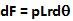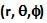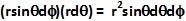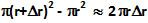## Tuesday, March 31, 2009

### Irodov Problem 1.291a)
The internal pressure p of the cylindrical tube pushes the walls of the cylinder normal to the surface of the cylinder (blue arrows) as shown in the figure. As a result it threatens to tear the cylinder apart into two halves. The net force exerted by the internal pressure on the walls has to be balanced by the stressin walls (thickness) of the cylinder (red arrows). The maximum stress that can be supported by the cylinder is the tensile strengthof the material. When the stress in the walls required to balance the net internal force on the walls becomes greater than the tensile strength of the material, the cylinder breaks apart.

If we cut the cylinder arbitrarily into two equal halves longitudinally, then the net force acting on the cylinder walls that opposes the stress is the component that is perpendicular to the plane of the cut. The effective area over which the pressure p acts is thus the cut plane ABCD with area 2rL and hence the total force exerted by the internal pressure is 2prL.

Another way of obtaining the same result is as follows: Consider an infinitesimally thin strip of length L on the cylinder surface that subtends an angle. The area of this strip will be. Consequently the force dF exerted on the strip will be. The component of the force that will be supported by the stress in the walls is. The net force that has to be supported by the stress in the walls of the cylinder can be obtained by integrating over the entire curved surface of the hemi-cylinder as,The total area that the stress acts on is the area of the thickness of the walls given by. Thus, the total force exerted by the stress in the walls is given by. In order for the cylinder not to tear apart the force due to pressure must be balanced by the force due to stress and so we have,b) The essential logic behind solving this problem is exactly the same as a) only the geometry is different. The net effective area over which the internal pressure acts in the hemi-sphere is the area of the great circle given by. The total force exerted by the internal pressure that opposes the stress in the walls is given by.

The same result can also be alternatively obtained as follows: Consider an infinitesimally small surface element that is located at spherical coordinates. The area of the surface element subtended by this infinitesimally small surface element is given by. The force dF exerted on this element if given by. The entire net force acting on the hemi-sphere is given by,The area over which the stress acts in the sphere is the area of the thickness of the walls given by. Thus, entire force exerted by the stress is given by. Since this must balance the force exerted on the walls by the internal pressure we have,